# The period T of a simple pendulum is the amount of time required for it to undergo one complete oscillation

The period T of a simple pendulum is the amount of time required for it to undergo one complete oscillation, if the length of the pendulum is L and the acceleration of gravity is g, then T is given by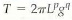Find the powers p and q required for dimensional consistency.

Time period of a simple pendulum can be defined as the amount of time required to complete one oscillation.
Equation for the time period of a simple pendulum can be expressed as follows: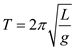Here, L is the length of the pendulum, and g is the acceleration due to gravity.

Calculate the powers p and q.
The equation is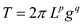…(1)
Carry out a dimensional analysis of T, L, and g.
Ignore the dimensions of 2◊ because it is a dimensionless quantity.Compare the above dimensional equation on both sides.
p + q = 0 …… (2)
And… (3)
Solve the above two equations.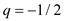Therefore the value of the power q is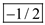.